Prof. Caine

•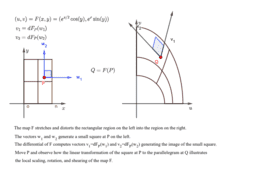The Differential of a Map

Activity

Prof. Caine

•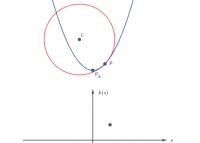Best Fitting Circle to a Parabola

Activity

Prof. Caine

•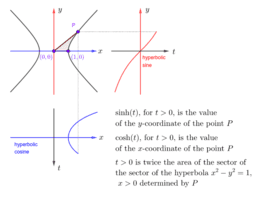Hyperbolic Sine and Cosine

Activity

Prof. Caine

•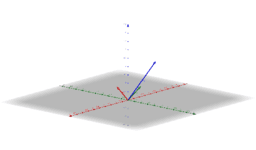Illustration of the Gram-Schmidt Process

Activity

Prof. Caine

•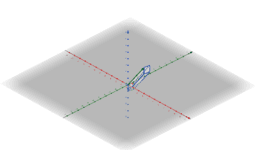Parallelopipeds for A-tI

Activity

Prof. Caine

•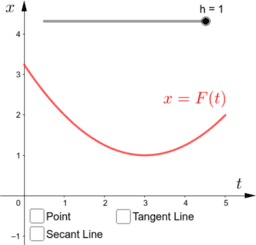The Big Ideas of Calculus I

Activity

Prof. Caine

•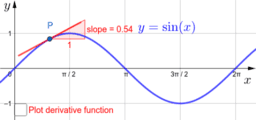Derivatives of Sine and Cosine

Activity

Prof. Caine

•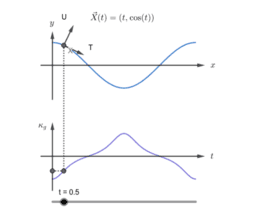Curvature function for the graph of cosine

Activity

Prof. Caine

•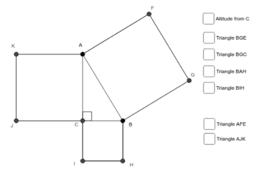Euclid's Proof of the Pythagorean Theorem

Activity

Prof. Caine

•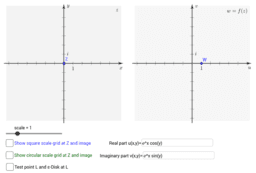Complex Functions as Mappings

Activity

Prof. Caine

•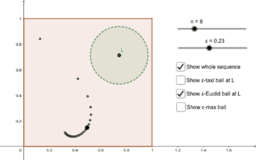Convergence in three metrics on the square

Activity

Prof. Caine

•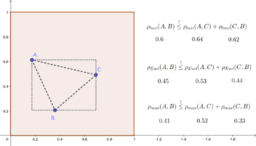Three metrics on the square

Activity

Prof. Caine

•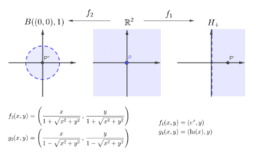The plane, the half-plane, and the disk are homeomorphic

Activity

Prof. Caine

•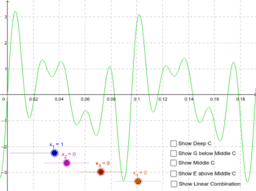C-major chord as a linear combination

Activity

Prof. Caine

•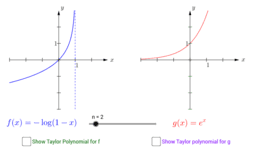Two Examples of Taylor Approximation

Activity

Prof. Caine

•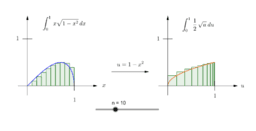Change of Variables

Activity

Prof. Caine

•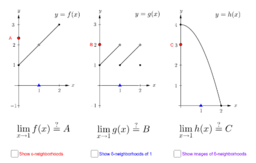Limits and Continuity

Activity

Prof. Caine

•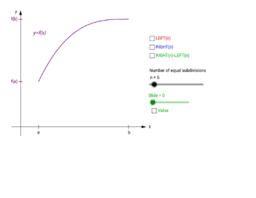The Sliding Boxes Proof

Activity

Prof. Caine

•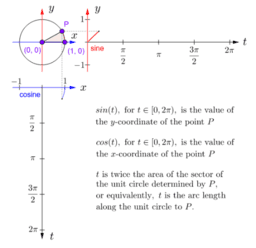Circular sine and cosine

Activity

Prof. Caine

•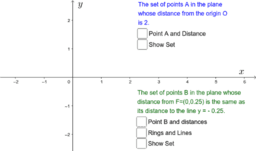Describing Sets in the Plane

Activity

Prof. Caine

•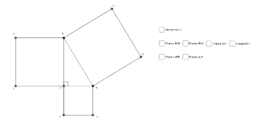Activity

Prof. Caine